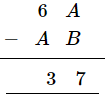# Replace A, B by suitable numerals.

Question:

Replace A, B by suitable numerals.Solution:

First look at the left column, which is:

$6-A=3$

This implies that the maximum value of A can be 3.

$A \leq 3$     ....(1)

The next column has the following:

$A-B=7$

To reconcile this with equation (1), borrowing is involved.

We know:

$12-5=7$

$\therefore A=2$ and $B=5$